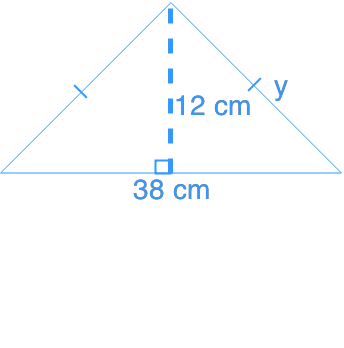# Using the pythagorean relationship

### Using the pythagorean relationship

In the previous section, we have learned the Pythagorean Theorem. We will now apply the knowledge to answer questions involving compound polygons.

#### Lessons

• Introduction
a)

i) What is Pythagorean Theorem?

ii) How to use Pythagorean Theorem to find sides of a triangle?

b)
What are Pythagorean Triples?

• 1.